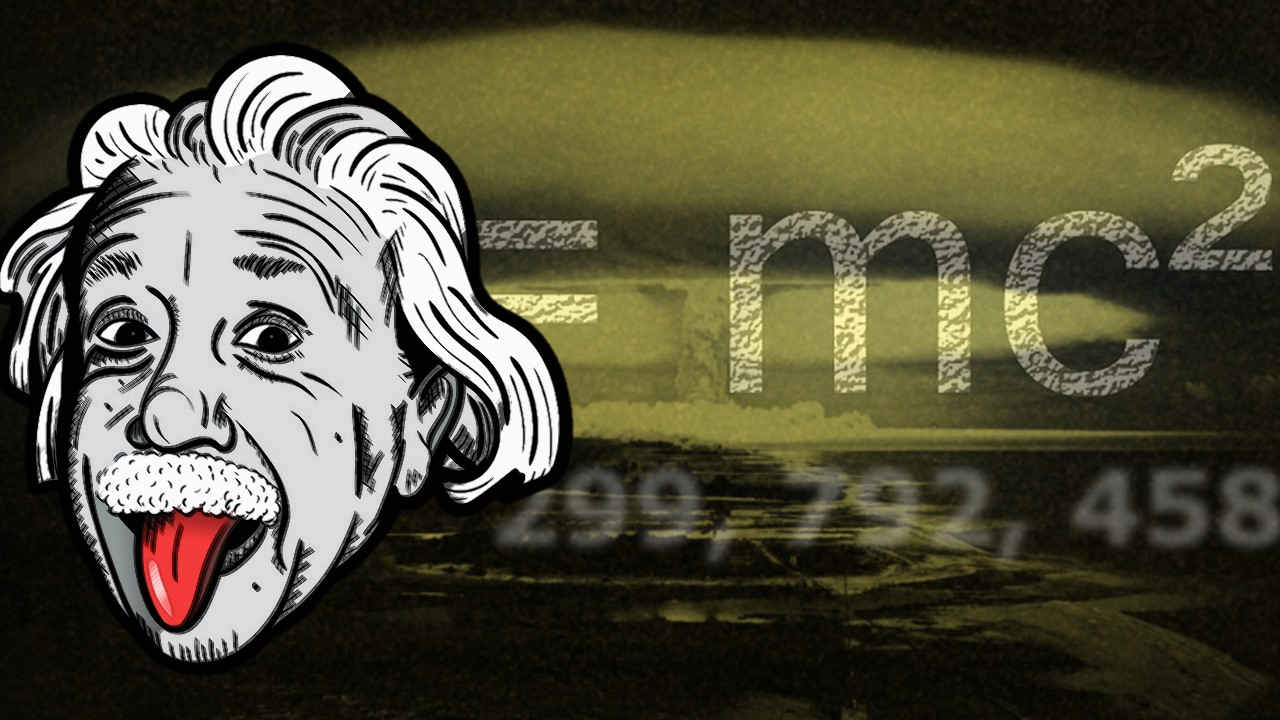# 5 Applications of Dimensional AnalysisThe concept of dimensional analysis was introduced by French mathematician Joseph Fourier in 1822. It is a useful method in physics and engineering to identify the relationships between various physical quantities by analyzing their base quantities.

There are seven base or fundamental quantities of primary importance in physics. The rest of all the physical quantities are derived, that is, written in terms of the following base quantities:

1. Mass (M)
2. Length (L)
3. Time (T)
4. Temperature (K)
5. Electric current (A)
6. Amount of substance (Mol)
7. Luminous intensity (Cd)
Others are derived quantities, for example: Speed is distance upon time, where distance is a type of length. So, the dimension of speed in terms of base quantities is [L][T]-1### 1. Find dimension of unknown quantity

Example: To increase the temperature of a substance by ΔT, heat required is given by Q=mSΔT where m is mass and S is specific heat capacity of the substance. We can find the dimension of S by doing simple algebra.

S=Q/mΔT

S=[M L2 T-2]/[M][K]

S=[L2 T-2 K-1]

Since heat (Q) is a type of energy we have used the dimension of energy [M L2 T-2]. Knowing the dimensional dependence is useful while performing experiment in laboratory.

### 2. Find unit of physical quantity

Example: The dimension of force is [M L T-2]. Therefore, the unit of force in the standard MKS system is kg.m.s-2 corresponding to the base quantities used. Likewise, the unit of force in the French CGS system is g.cm.s-2

### 3. To check correctness of formula

In an equation, the left hand side should match the dimensions on the right hand side. That's because, you can't have apples on one side and oranges on the other. This is useful in multiple choice questions as it helps in eliminating the wrong options.

Example: Is force F=mv2/r correct? where m is mass, v is velocity and r is radius. To find out, we start by writing the dimensions on both sides.

[M L T-2] = [M][LT-1]2/[L]

[M L T-2] = [M][L2T-2][L-1]

∴ [M L T-2] = [M][L][T-2]

The formula is correct. In fact, it is the centripetal force if you might recall, which acts upon a body directed towards a fixed center, thus keeping it in orbit.

### 4. To roughly derive formula

Example: Suppose that the time period of a simple pendulum is dependent on mass of the bob, length of the string and gravitational acceleration due to earth.Let's assume that the time period is proportional to some powers of mass, length and gravity: t∝malbgc

To find the formula of time period, we must obtain values for a, b and c. We start by writing the dimensions on both sides.

[M]0[L]0[T] = (constant)[M]a[L]b[LT-2]c

The dimension of constant is 1. So,

[M]0[L]0[T] = [M]a[L]b[LT-2]c

Comparing the exponents on both sides, we get:

a=0, b+c=0 and -2c=1

On solving we obtain values of a=0, b=1/2 and c=-1/2. Now, putting back in the original equation t∝malbg gives:

∝ m0l1/2g-1/2

t=(constant) l1/2g-1/2

Thus, time period of a simple pendulum is independent of mass of the bob. This is because, physically speaking, mass is both the cause of swinging motion and the resistance to swinging motion, so it cancels out.

### 5. To express in new base quantities

This type of questions can come up in competitive exams like the JEE-Main. Example: If pressure, velocity and time are taken as base quantities, then what would be the dimension of force?

Let's start by assuming powers

Force = Pavbtc

And write dimensions on both sides

[M L T-2] = [ML-1T-2]a[LT-1]b[T]c

[M L T-2] = [M]a[L]-a+b[T]-2a-b+c

Comparing powers on both sides

a=1, -a+b=1 ⇒ b=2 and -2a-b+c=-2 ⇒ c=2

∴ Force = Pv2t2